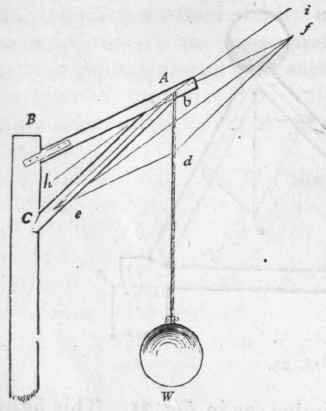Figs. 26 and 27 exhibit two methods of supporting the equal weights, W and W. Let it be required to measure and compare the strains produced on the pieces, A B and A C. Construct the parallelogram of forces, ebfd, according to Art. 71. Then bf will show the strain on A B, and b e the strain on A C. By comparing the figures, bd being equal in each, it will be seen that the strains in Fig. 26 are about three times as great as those in tion of forces; and the two are founded on one and the same principle, and may be solved in the same manner. For example, let A and B (Fig. 28) be two pieces of timber, pressed in the direction of their length towards b - A by a force equal to 6 tons weight, and B 9 tons. To find the direction and amount of pressure they would unitedly exert, draw the lines b e and b f in a line with the axes of the timbers, and make b e equal to the pressure exerted by B, viz., 9; also make b f equal to the pressure on A, viz., 6, and complete the parallelogram of forces ebfd; then bd, the diagonal of the parallelogram, will be the direction, and its length, 9.25, will be the amount, of the united pressures of A and of B. The line b d is termed the resultant of the two forces bf and be. If A and B are to be supported by one post, C, the best position for that post will be in the direction of the diagonal bd; and it will require to be sufficiently strong to support the united pressures of A and of B, which are equal to 9-25 or 9 1/4 tons.Fig. 26.Fig. 27.

Fig. 27; the position of the pieces, A B and A C, in Fig. 27, is therefore far preferable.Fig. 28.

80. - The Composition of Forces: consists in ascertaining the direction and amount of one force which shall be just capable of balancing two or more given forces, acting in different directions. This is only the reverse of the resolu-

##### Strains Involved In CraneFig. 29.

81. - Another Example - Let Fig. 29 represent a piece of Laming commonly called a crane, which is used for hoisting heavy weights by means of the rope, B bf, which passes over a pulley at b. This, though similar to Figs. 26 and 27, is, however, still materially different. In those figures, the strain is in one direction only, viz., from b to d; but in this there are two strains, from A to B and from A to W. The strain in the direction A B is evidently equal to that in the direction A W. To ascertain the best position for the strut A C, make b e equal to bf, and complete the parallelogram of forces ebfd; then draw the diagonal bd, and it will be the position required. Should the foot, C, of the strut be placed either higher or lower, the strain on A C would be increased. In constructing cranes, it is advisable, in order that the piece B A may be under a gentle pressure, to place the foot of the strut a trifle lower than where the diagonal bd would indicate, but never higher.Fig. 30.